### Home > PC > Chapter 4 > Lesson 4.1.4 > Problem4-56

4-56.
1. Take the reciprocal of each of the following fractions and then simplify. Homework Help ✎

1.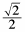2.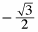3. 0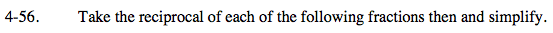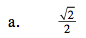$\frac{2}{\sqrt{2}}=\frac{2}{\sqrt{2}}\cdot \frac{\sqrt{2}}{\sqrt{2}}$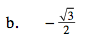Follow similar steps as shown in part (a).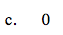What is the value of 1/0?